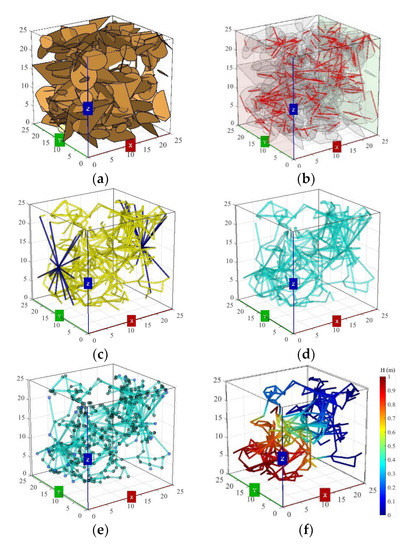Previous Article in Journal
Placed Riprap Deformation Related to Axial Load at Toe Support: Physical Modelling

Article

# An Equivalent Pipe Network Modeling Approach for Characterizing Fluid Flow through Three-Dimensional Fracture Networks: Verification and Applications

State Key Laboratory for Geomechanics and Deep Underground Engineering, China University of Mining and Technology, Xuzhou 221116, China
*
Author to whom correspondence should be addressed.
Water 2022, 14(10), 1582; https://doi.org/10.3390/w14101582
Received: 11 April 2022 / Revised: 3 May 2022 / Accepted: 9 May 2022 / Published: 16 May 2022
The equivalent pipe network (EPN) model is an effective way to model fluid flow in large-scale fractured rock masses with a complex fracture network due to its straightforwardness and computational efficiency. This study presents the EPN model for characterizing fluid flow through three-dimensional fracture networks using the Monte-Carlo method. The EPN model is extracted from an original three-dimensional discrete fracture network (DFN) model and is used to simulate the fluid flow processes. The validity of the proposed EPN modeling approach is verified via the comparisons of permeability (k) with analytical solutions and simulation results reported in the literature. The results show that the numerically calculated k using EPN models agrees well with the analytical values of simplified DFN models and the simulation results of complex DFN models. The k increases following an exponential function with the increment of mean length of exponentially distributed fractures (u), which is strongly correlated with fracture density (P32) and average intersection length (Li). The P32 increases in an exponential way with the increment of u. The Li increases as u increases, following a power-law function. The increment of u leads to the increment of a number of long fractures in three-dimensional DFN models. A larger u results in a denser fracture network and a stronger conductivity when the number and length distribution range of fractures remain the same. The representative elementary volumes (REVs) of three-dimensional DFN models with u = 9 m and P32 = 0.4 m2/m3 are determined as 2.36 × 104 m3, 9.16 × 103 m3, and 1.26 × 104 m3 in 3 flow directions, respectively. View Full-Text
Show FiguresFigure 1

MDPI and ACS Style

Zhang, J.; Liu, R.; Yu, L.; Li, S.; Wang, X.; Liu, D. An Equivalent Pipe Network Modeling Approach for Characterizing Fluid Flow through Three-Dimensional Fracture Networks: Verification and Applications. Water 2022, 14, 1582. https://doi.org/10.3390/w14101582

AMA Style

Zhang J, Liu R, Yu L, Li S, Wang X, Liu D. An Equivalent Pipe Network Modeling Approach for Characterizing Fluid Flow through Three-Dimensional Fracture Networks: Verification and Applications. Water. 2022; 14(10):1582. https://doi.org/10.3390/w14101582

Chicago/Turabian Style

Zhang, Jing, Richeng Liu, Liyuan Yu, Shuchen Li, Xiaolin Wang, and Ding Liu. 2022. "An Equivalent Pipe Network Modeling Approach for Characterizing Fluid Flow through Three-Dimensional Fracture Networks: Verification and Applications" Water 14, no. 10: 1582. https://doi.org/10.3390/w14101582

Find Other Styles
Note that from the first issue of 2016, MDPI journals use article numbers instead of page numbers. See further details here.

1